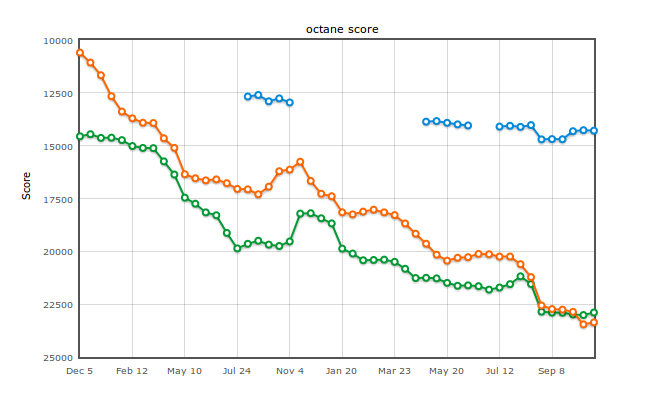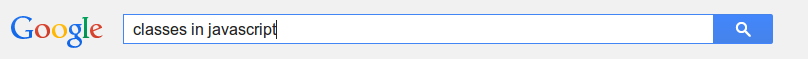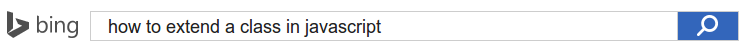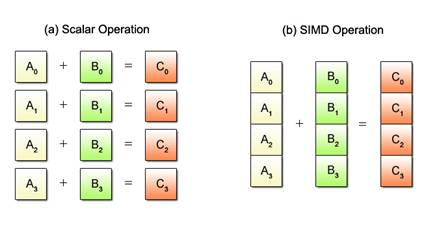# The future of Javascript.

## Benjamin BouvierJS DevPlatform engineer on JS engine.Maintainer of AreWeFastYet.com
h4writer.com
@h4writer*not an official logo (credits to Steven Wittens)
@njbenji

# EcmaScript 6.0

• ## ES6: Promises

June 2015

Browsers eagerly implemented stuffTraceur6to5

# ES6: Generators

Generate a sequence, one item at a time

1 1 2 3 5 8F

2

D

A

Z

U

C

3

1

8

3

9

1

More general: Function that can be paused in the middle

## Syntax

Star: indicates a generator function

``````function *foo() {
// ...
yield "bar1";
// ...
yield "bar2";
// ...
}``````

Yield: pauses the function

## Usage

``````function *foo() {
console.log("running");
}

foo();``````

Nothing happens!

``var generator = foo();``
``// generator = { next: function() { ... }, throw: function() { ... }};``

## Usage

``````function *foo() {
console.log("running");
var x = yield 1;
var y = yield 2;
return 3;
}

var generator = foo();

var result1 = generator.next();
// console: "running"
// result1 = {value: 1, done: false}

var result2 = generator.next();
// result2 = {value: 2, done: false}

var result3 = generator.next();
// result3 = {value: 3, done: true}``````

## Usage

``````function *foo() {
var x = yield 1;
var y = yield 2;
return x + y;
}

var generator = foo();

var result1 = generator.next();
// result1 = {value: 1, done: false}

var result2 = generator.next(10);
// result2 = {value: 2, done: false}

var result3 = generator.next(20);
// result3 = {value: 30, done: true}``````

### Example 1: Iteration

``````function *range(start, end) {
while (start != end) {
yield start;
start++;
}
}``````

#### Usage

``````for (let i of range(0, 10)) {
console.log(i);
}``````

#### Result

``````> 0
> 1
> 2
> 3
> 4
> 5
> 6
> 7
> 8
> 9
``````

### Example 2: Fibonacci

``````function *fibonacci() {
var prev = 0, cur = 1;
while (true) {
let sum = cur + prev;
yield sum;
prev = cur
cur = sum
}
}``````

#### Usage

``````for (let n of fibonacci()) {
if (n > 1000)
break;
console.log(n);
}``````

#### Result

``````> 0
> 1
> 1
> 2
> 3
> 5
> 8
...
``````

### Generators: Compatibility2639n/a26n/a Released 14 months ago Released 3 months ago Released 2 months ago

# ES6: Classes## Prototype based !!

``````function Animal() {
this.cuteness = 0; // neutral
this.hunger = 100; // 0: has no hunger, 100: needs food
this.approach = function() { } // do nothing
// Getters
Object.defineProperty(this, "fullness", {
get: function() {
return 100 - this.hunger;
}
});
}
Animal.prototype.feed = function(type) {
this.hunger--;
}
Animal.talk = function(animal1, animal2) {
// when animals are same species chitchat else growl
}
var unknownSpecies = new Animal();
``````

Why can't we use classes. I know classes.What is this?## Syntax

``````class Animal() {
constructor() {
this.cuteness = 0; // neutral
this.hunger = 100; // 0: has no hunger, 100: needs food
}
approach() {
// do nothing
}
get fullness() {
return 100 - this.hunger;
}
feed(type) {
this.hunger--;
}
static talk(animal1, animal2) {
// when animals are same species chitchat else growl
}
}
``````

A minimal class proposal

## Inheritance

``````class Cat extends Animal {
constructor() {
super();
}
approach() {
this.runAway()
}
feed(type) {
if (isDelicious(type))
super.feed(type)
// Cats are picky even when hungry.
}
}
``````

No multiple inheritance!

### Classes: Compatibilityn/an/an/an/an/a Available in "Technical Preview" using "Experimental Web Platform Features"Traceur6to5

# ES6: Arrow functions

## Problem: |this| is dynamic scoped

``````function Archer() {
this.arrows = 100;

setInterval(function shoot() {
this.arrows--;
}, 5 * 1000);
}

var a1 = new Archer();``````

## Solution 1: lexical alias to |this|

``````function Archer() {
var self = this;
this.arrows = 100;

setInterval(function shoot() {
self.arrows--;
}, 5 * 1000);
}

var a1 = new Archer();``````

## Solution 2: bind the callback function

``````function Archer() {
this.arrows = 100;

setInterval(function shoot() {
this.arrows--;
}.bind(this), 5 * 1000);
}

var a1 = new Archer();``````

## New solution: arrow functions

``````function Archer() {
this.arrows = 100;

setInterval(() => {
this.arrows--;
}, 5 * 1000);
}

var a1 = new Archer();``````

Shorter syntax

Lexically binds |this|

## Syntax: arrow functions

``````([param] [, param]) => {
statements
}

param => expression
``````

## Examples

``````// 1. Basic
(x, y) => {
return x+y
}

// 2. No return needed (for single expressions)
(x, y) => x+y

// 3. No parentheses (for one argument)
x => Math.pow(x, 2);

// 4. Or
x => {
return Math.pow(x, 2);
}

// 5. No arguments
() => Math.random()

``````

### Arrow functions: Compatibility23n/an/an/an/a Released one and a half year ago Available in "Technical Preview" using "Experimental Web Platform Features"

# ES6: Destructuring

Assign data from Array or Object to variables

## Destructuring: List matching.

``````var [a, b] = [1, 2];
// a = 1, b = 2

var [a, ,b] = [1,2,3];
// a = 1, b = 3

var [a, b, ...c] = [1, 2, 3, 4, 5];
// a = 1, b = 2, c = [3, 4, 5]

var [b, a] = [a, b]
// swaps the content of a and b``````

## Destructuring: Object matching.

``````var { data : var1 } = { data : 50 }
// var1 = 50

var { m: month, y: year } = { d: 1, m: 2, y: 2015}
// month = 2, year = 2015

var { child: { data: element  } } = { child : { child: null, data: 1}, data: null }
// element = 1;

var { data : data } = { data : 50 }
var { data } = { data : 50 }
// data = 50

var { rhs, lhs, data } = { rhs : {}, lhs: {}, data: 50 }
// rhs = {}, lhs = {}, data = 50``````

## Destructuring: Useable in parameter position.

``````function g({name: x}) {
console.log(x);
}
g({name: 5, foo: 0})

// Console: 5``````

## Destructuring: Using defaults.

``````// Fail-soft destructuring
var [a] = [];
a === undefined;

// Fail-soft destructuring with defaults
var [a = 1] = [];
a === 1;``````

Not implemented yet!

### Destructuring: Compatibility34n/an/an/a7.1 Released 2 months ago Released 5 monts ago

# ES6: Modules

Fix dependencies of Libraries

Using the 'script'-tag is not scalable

CommonJS Modules
Asynchronous Module Definition (AMD)

## Module pattern

``````/* ------ lib.js ------ */
var privateProperty = 1;
export var publicProperty = 2

function privateFunction() { /* ... */ }
export function publicFunction() { /* ... */ }

export default function () { /*  */ }

/* ------ main.js ------ */
import { publicProperty, publicFunction } from 'lib';
// publicProperty = 2, publicFunction = function () { /* ... */ }

import { publicFunction as libfunc } from 'lib';
// libfunc = function() { /* ... */ }

import { default as foo } from 'lib';
import foo from 'lib';
// foo now contains the default function

import * as mylib from 'lib';
// mylib = { publicProperty: 2, publicFunction: function() { /* ... */}}
``````

``````System.import('some_module')
// Returns a promise? that loads the module async``````

To be determined

Make it possible to load libraries sync and async

# ES6: Promises

Abstraction for Async code

## Problem

JS executes sequentially and blocks all other execution

``````while (true) {
// Crunch some data.
}````````````// Make sure first image is loaded
var img = document.getElementsByTagName("img")
while (!img.complete) {
// not yet completed
}
// Completed, now we can do something with it.``````

So we need async behaviour.

Enter events and continuation functions

``````// Make sure first image is loaded
var img = document.getElementsByTagName("img")
// Completed, now we can do something with it.
}``````

Just add some more code. DUH!

``````if (img.completed) {
}``````

## Promises

the idea is to make this simpler with promises and have some nice tools around it.

## Syntax

``````
function executor(resolve, reject) {
/* do something */

if (/* fail */)
reject(); // Bonus, will also get called upon Error.

/* done */
resolve();
}

var promise = new Promise(executor);

promise.then(function() {
/* Let's do something with the result. */
}, function() {
/* Failed. */
})
``````

``````var img = document.getElementsByTagName("img")
// Completed, now we can do something with it.
})``````

## Promise Example: Chaining

``````var imgs = document.getElementsByTagName("img")
}).then(function() {
});``````

## Example: Parallel testing of multiple promises

``````var imgs = document.getElementsByTagName("img")
Promise.all(promises).then(function() {
})``````

### Promises: Compatibility2932n/a197.1 Released 9 months ago Released 1 year ago Available in "Technical Preview" using "Experimental Web Platform Features" Released 1 year ago Released 5 monts ago

# The Future

• ## SIMD.js

Might be present in ES7, or even later.

Very experimental features (implemented in Nightly).

Instable APIs.

# Typed Objects

Add a way to describe data structures which members have defined types.

Types among {u,}int{8,16,32,64} / float{32,64} / String / Object, etc..

``````
var {StructType, ArrayType} = TypedObject;
// Create a simple type
var Pixel = new StructType({'r': uint8, 'g': uint8, 'b': uint8});
``````
``````
// Create an array type
var Square = new ArrayType(Pixel, 4);
``````
``````
// Compose types!
var Image = new StructType({'id': uint16, 'src': Square});
``````

## Create your own typed objects!

``````
var p = new Pixel({r: 128, g: 42, b: 25});
p.g; // shows 42
``````
``````
// Omit a field in the constructor to give it a default value
var p2 = new Pixel({r: 128, b: 56});
p2.g; // shows 0
``````
``````
// Arguments are coerced to fit the specified type
var p3 = new Pixel({r: 128, g: 35, b: 1337});
p2.b; // shows 57
``````
``````
// Let's instantiate an ArrayType
var sq = new Square([
{r: 24, g: 35, b: 46},
{r: 52, g: 63, b: 74},
p,
p2
]);
``````

## Features of typed objects

``````
// You can't add attributes to Typed objects instances
var p = new Pixel();
p.id = "let's sneak in a string, i'm sure the VM won't see it";
// TypeError: Pixel is not extensible
``````
``````
// Typed objects are objects (!)
typeof p2; // "object"

var p2 = new Pixel();
p == p2; // false
p === p2; // false
``````

## 5 Reasons To Love Typed Objects; #5 Is My Favourite

• Better memory layout.
• No deoptimizations due to shapes changing.
• Predictable performance.
• ... and it can inspire optimizations in your VM today!

## Can we remove boxing of plain JS objects?

If we can prove an object has a stable shape ("hidden class") and we know its definite properties, we can handle it as a Typed Object.

``````
function PixelFactory(r, g, b) {
this.r = r | 0; // Forces int32 coercion
this.g = g | 0;
this.b = b | 0;
}
``````
``````
var Pixel = new StructType({r: int32, g: int32, b: int32});
// What if we handle an object created from the PixelFactory as a
// Typed Object with the Pixel type?
``````

# SIMD.js

Use vector operations directly in JavaScript and have these jitted as vector processor instructions.

## Single Instruction, Multiple DataA normal operation takes two input operands.

A SIMD operation operates on pairs of input operands.

Implementations of SIMD: SSE (x86), NEON (arm)

This is fast! Mostly used in heavily parallel processing (audio / image processing, codecs, games...).

## The SIMD.js model

Fixed-length: 128 bits (much useful, well supported, very SIMD, wow).

Uniform behaviours of operation accross platforms.

New value types: int8x16, int16x8, int32x4, float32x4, float64x2.

These can be embedded in Typed Objects descriptors.

## Available operations

• - Basic arithmetic (add, sub, mul, div, abs, sqrt, min/max, etc.).
• - Comparisons (lessThan{,OrEqual}, greaterThan{,OrEqual}, equal, notEqual
• - Conversions and bitcasts (e.g., float32x4.fromInt32x4, float32x4.fromInt32x4Bits, etc.)
• - Bitwise operations (or, and, xor, not, shiftLeftByScalar, shiftRightLogicalByScalar, shiftRightLogicalByScalar, etc.)
• - Manipulate data (lane accessors and setters, select, bitselect, shuffles, partial or full loads and stores within typed arrays)

## can haz code? okthxbye

``````
function fibonacci4(n) {
var x = SIMD.int32x4(1, 1, 2, 3);
var y = SIMD.int32x4(1, 2, 3, 5);

while (n--) {
var tmp = y;
x = tmp;
}

return x;
}

var v = fibonacci4(10);
console.log(v.x, v.y, v.z, v.w); // (89, 144, 233, 377)
``````

## Another example

``````
var x = SIMD.float32x4(1, 2, 3, 4);
var y = SIMD.float32x4(1, 2, 3, 4);

var previous = SIMD.float32x4.splat(0); // copies the value in all four lanes

var threshold = SIMD.float32x4.splat(.001337);

while (true) {
previous = x;

x = SIMD.float32x4.sqrt(x); // square root

let v = SIMD.float32x4.sub(x, previous); // subtract
v = SIMD.float32x4.abs(v); // absolute

let cmp = SIMD.float32x4.lessThanOrEqual(v, threshold); // <=
if (cmp.signMask == 0b1111) // true in all lanes
break;
};

console.log(x.x, x.y, x.z, x.w);
``````